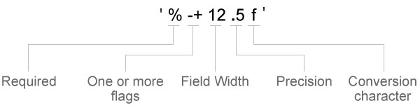# pie

## 语法

``pie(X)``
``pie(X,explode)``
``pie(X,labels)``
``pie(X,explode,labels)``
``pie(ax,___)``
``p = pie(___)``

## 说明

``pie(X)` 使用 `X` 中的数据绘制饼图。饼图的每个扇区代表 `X` 中的一个元素。如果 `sum(X) ≤ 1`，`X` 中的值直接指定饼图扇区的面积。如果 `sum(X) < 1`，`pie` 仅绘制部分饼图。如果 `sum(X) > 1`，则 `pie` 通过 `X/sum(X)` 对值进行归一化，以确定饼图的每个扇区的面积。如果 `X` 为 `categorical` 数据类型，则扇区对应于类别。每个扇区的面积是类别中的元素数除以 `X` 中的元素数的结果。`

``pie(X,explode)` 将扇区从饼图偏移一定位置。`explode` 是一个由与 `X` 对应的零值和非零值组成的向量或矩阵。`pie` 函数仅将对应于 `explode` 中的非零元素的扇区偏移一定的位置。如果 `X` 为 `categorical` 数据类型，则 `explode` 可以是由对应于类别的零值和非零值组成的向量，或者是由要偏移的类别名称组成的元胞数组。`

``pie(X,labels)` 指定用于标注饼图扇区的选项。在本例中，`X` 必须为数值。`

``pie(X,explode,labels)` 偏移扇区并指定文本标签。`X` 可以是数值或分类数据类型。`

``pie(ax,___)` 将图形绘制到 `ax` 指定的坐标区中，而不是当前坐标区 (`gca`) 中。选项 `ax` 可以位于前面的语法中的任何输入参数组合之前。`

``p = pie(___)` 返回一个由补片和文本图形对象组成的向量。该输入可以是先前语法中的任意输入参数组合。`

## 示例

```X = [1 3 0.5 2.5 2]; pie(X)``````explode = [0 1 0 1 0]; pie(X,explode)``````X = 1:3; labels = {'Taxes','Expenses','Profit'}; pie(X,labels)``````X = 1:3; labels = {'Taxes','Expenses','Profit'}; p = pie(X,labels)``````p = 1x6 graphics array: Patch Text Patch Text Patch Text ```

```t = p(6); t.BackgroundColor = 'cyan'; t.EdgeColor = 'red'; t.FontSize = 14;``````X = [1/3 2/3]; pie(X,'%.3f%%')``````X = [0.19 0.22 0.41]; pie(X)``````y2010 = [50 0 100 95]; y2011 = [65 22 97 120]; labels = {'Investments','Cash','Operations','Sales'};```

```t = tiledlayout(1,2,'TileSpacing','compact'); % Create pie charts ax1 = nexttile; pie(ax1,y2010) title('2010') ax2 = nexttile; pie(ax2,y2011) title('2011') % Create legend lgd = legend(labels); lgd.Layout.Tile = 'east';``````X = categorical({'North','South','North','East','South','West'}); explode = {'North','South'}; pie(X,explode)``````explode = [0 1 1 0]; pie(X,explode)``````X = categorical({'North','South','North','East','South','West'}); explode = {}; labels = {'E','N','S','W'}; pie(X,explode,labels)``````X = categorical({'North','South','North','East','South','West'}); explode = {'West'}; labels = {'E','N','S','W'}; pie(X,explode,labels)```## 输入参数

• 如果 `X` 为数值，则 `X` 中的所有值必须为有限值。

• 如果 `X` 是分类数组，则 `pie` 将忽略未定义的元素。

• 如果 `X` 为数值，则 `explode` 必须是逻辑向量或数值向量，或由对应于 `X` 的零或非零值组成的矩阵。一个真（非零）值从饼图中心将相应的扇区偏移一定位置，这样如果 `explode(i,j)` 是非零值，则 `X(i,j)` 将从中心偏移。`explode``X` 的大小必须相同。

• 如果 `X` 是分类数组，则 `explode` 可以是由类别名称组成的字符向量元胞数组或字符串数组。`pie` 将与 `explode` 中的类别对应的扇区偏移一定的位置。

• 如果 `X` 是分类数组，`explode` 也可以是逻辑向量或数值向量，其中包含与 `X` 中的每个类别对应的元素。`pie` 函数按类别顺序将对应于 true（非零）的扇区偏移一定的位置。

#### 显示文本标签

`X` 包含数值时，`labels` 中的元素数必须等于 `X` 中的元素数。

`X` 包含分类值时，`labels` 中元素的数量和顺序必须等同于 `X` 中类别的数量和顺序。要确定类别的数量和顺序，请使用 `categories` 函数。

#### 以特定格式显示百分比1. 一个或多个标志 - 用于显示加号、小数点和调整标签的选项。

• 加号 (`+`) - 在正值旁边显示加号。

• 井号 (`#`) - 显示小数点，即使精度为 `0` 也是如此，例如 `'50.'`

• 减号 (`–`) - 左对齐标签，并用空格填充标签的末尾，而不是在开头用空格填充。

2. 字段宽度 - 标签中要显示的最小字符数。请将字段宽度指定为整数值。如果标签中的位数小于字段宽度，则在标签上用空格填充。

3. 精度 - 小数点右侧的位数。将精度指定为小数点后跟一个整数值。

4. 转换字符 - 定点或指数记数法。有关转换字符的列表，请参阅下表。如果您指定的转换字符不适合数据，MATLAB 将改用 `%e`

`f`定点记数法。精度值指示小数位数。`'%.4f%%'` 将占饼图 6.25% 的扇区的标签显示为 `6.2500%`
`e`指数记数法。精度值指示小数位数。`'%.4e%%'` 将占饼图 6.25% 的扇区的标签显示为 `6.2500e+00%`
`g`更精简版本的 `e``f`，不带尾随零。精度值指示最大小数位数。`'%.4g%%'` 将占饼图 6.25% 的扇区的标签显示为 `6.25%`

## 输出参数

`Patch``Text` 对象，以向量形式返回。有关详细信息，请参阅 Patch 属性Text 属性

R2019b 中的行为有变化